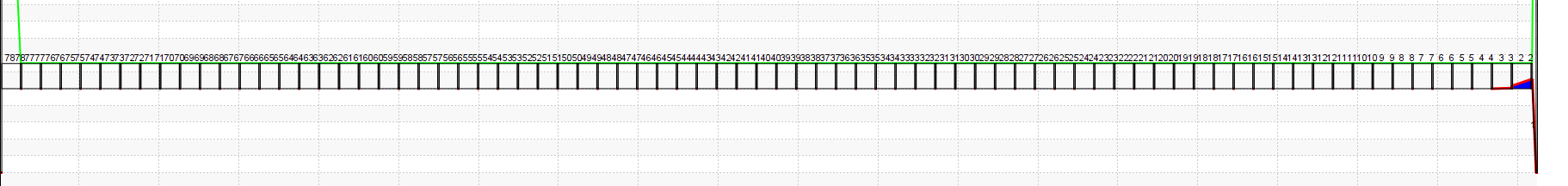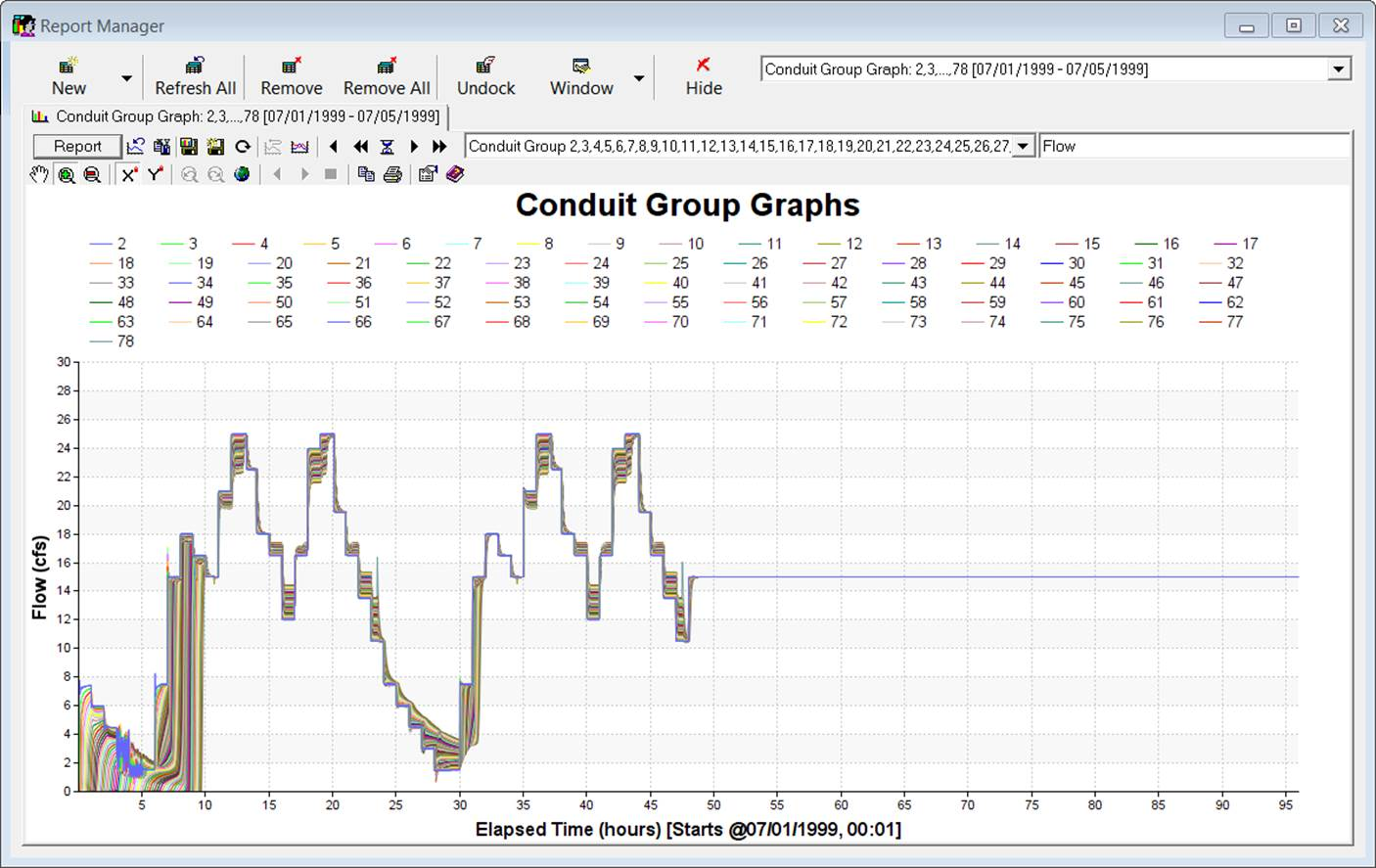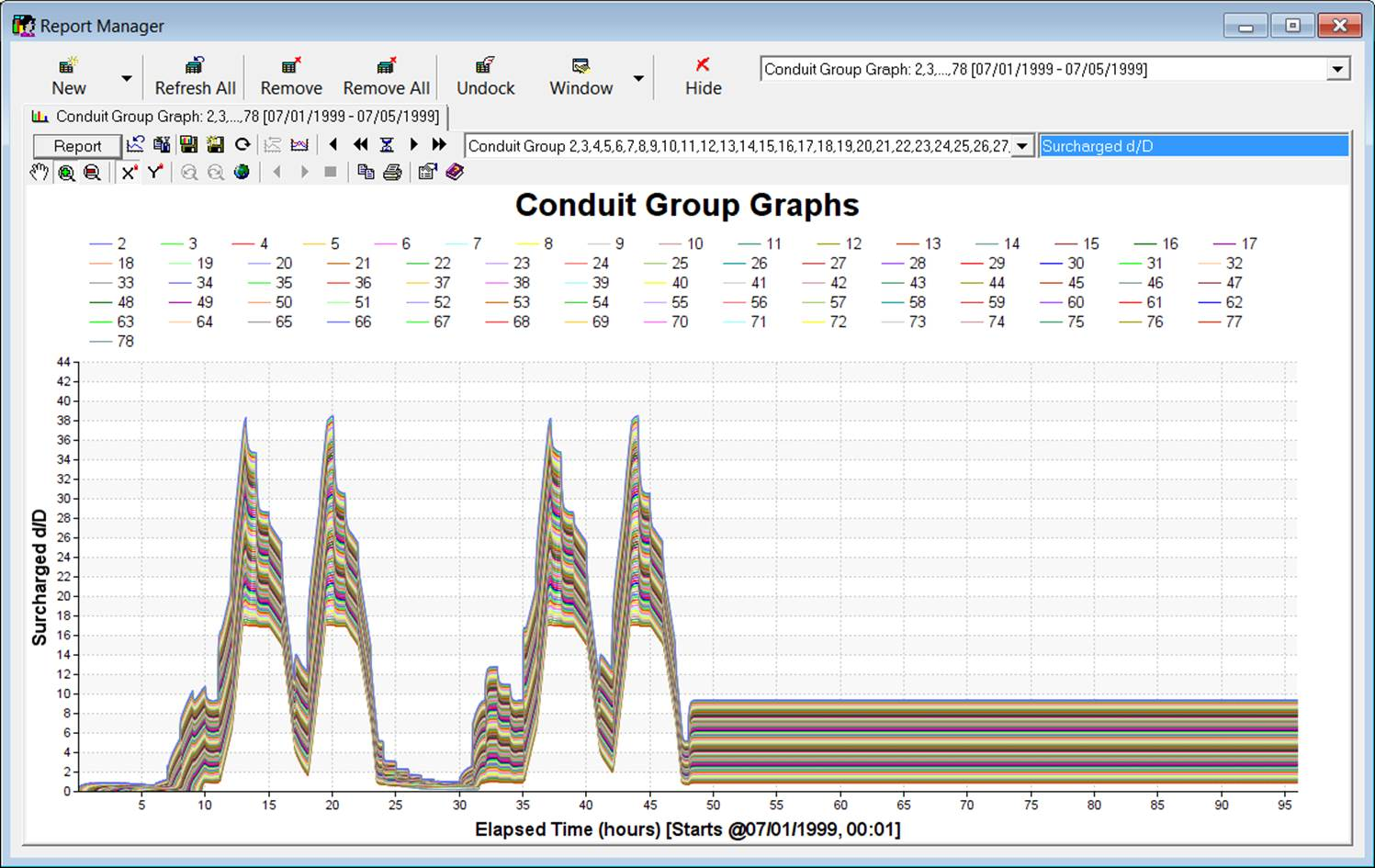#INFOSWMM

# A Visual Look at a Series of HW Force Mains in #InfoSWMM and #SWMM5

A Visual Look at a series of Hazen Williams friction loss HW Force Mains in #InfoSWMM and #SWMM5.  All of these graphs were made in the advanced graphical tools of InfoSWMM.

The figures show the progress of the water downstream along with comments on individual terms. Here is the  list of the figures

Figure 1 - All of the Force Mains (FM) use Hazen Williams (HW) friction loss (C of 100), are 3 feet in diameter, flat and 500 feet long). The Manning's n value for partial flow is 0.013

Figure 2 – The upstream pump flow rates in the long Force Main Model is a Volume/Flow Pump Type with these Pump Flows over time.

Figure 3 – Speed Ratio of the Pump over time. The Speed Ratio changes as the DWF changes.

Figure 4 – Mean flow in CFS and the Pump Pattern over time.

Figure 5 – The Flow in the pump has a maximum of 25 cfs.

Figure 6 – Flows in many connected Force Mains (FM) using HW friction loss over time reacting to the Turn on and Turn off’s of a Pump. Thee flow is attenuated as you move downstream.

Figure 7 – The Depths in many connected Force Mains (FM) using HW friction loss over time reacting to the Turn on and Turn off’s of a Pump. HW is used for full pipes and Manning’s n for partially full pipes.

Figure 8 – The capacity in the pipe is based on the current cross sectional area / full flow area and not depth. It is calculated at the middle of the link.

Figure 9 – The Surcharged d/D is based on the average of the depths at both ends of the link and can range from 0 to a large number.

Figure 10 – The flow volume over time - there is no increase in volume when the pipe is full as the SWMM5 solution uses a point iteration solution at the node to make the sum of the flows zero.

Figure 11 – The HGL in many connected Force Mains (FM) using HW friction loss over time reacting to the Turn on and Turn off’s of a Pump

Figure 12 – The Froude Number is zero when the pipe is full as the non linear term in the St Venant Equation is zero.

Figure 13 - The top width of the pipe over time - a full pipe is 3 feet wide.

Figure 14 – HGL of Modeled or Modelled FM and Pump System.Figure 1 - All of the Force Mains (FM) use Hazen Williams (HW) friction loss (C of 100), are 3 feet in diameter, flat and 500 feet long). The Manning's n value for partial flow is 0.013Figure 2 – The upstream pump flow rates in the long Force Main Model is a Volume/Flow Pump Type with these Pump Flows over time.Figure 3 – Speed Ratio of the Pump over time. The Speed Ratio changes as the DWF changes.Figure 6 – Flows in many connected Force Mains (FM) using HW friction loss over time reacting to the Turn on and Turn off’s of a Pump. Thee flow is attenuated as you move downstream.Figure 7 – The Depths in many connected Force Mains (FM) using HW friction loss over time reacting to the Turn on and Turn off’s of a Pump. HW is used for full pipes and Manning’s n for partially full pipes.Figure 8 – The capacity in the pipe is based on the current cross sectional area / full flow area and not depth. It is calculated at the middle of the link.Figure 9 – The Surcharged d/D is based on the average of the depths at both ends of the link and can range from 0 to a large number.Figure 10 – The flow volume over time - there is no increase in volume when the pipe is full as the SWMM5 solution uses a point iteration solution at the node to make the sum of the flows zero.Figure 11 – The HGL in many connected Force Mains (FM) using HW friction loss over time reacting to the Turn on and Turn off’s of a PumpFigure 12 – The Froude Number is zero when the pipe is full as the non linear term in the St Venat Equation is zero.